# Linear Equation

Lets consider two linear equations that we want to find a solution for.
One way to find a solution is to use the Solver add-in.
This add-in allows you to use a formula and specify a result in order to determine the necessary argument values.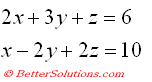### Create a simple Table

You must first create a simple worksheet that contains the following information.
The corresponding formulas are entered into

Create the following named ranges:
"C7" = Xvalue
"C8" = Yvalue
"C9" = Zvalue
The formula corresponding to the first equation is entered into cell "C3" using the values from the above named ranges.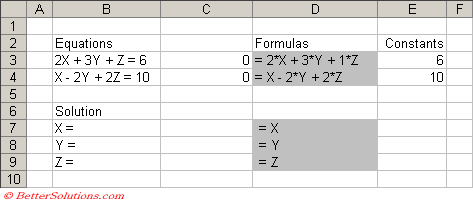Select (Tools > Solver) to display the Solver dialog box.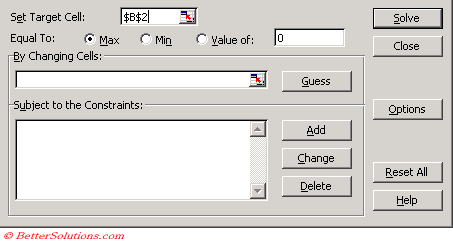To calculate the desired result Excel will change the underlying values in your cells.
When the Solver finds a solution you can either apply the new cell values or return to the original values.
Click on the Solve button after a second or two Solver will report if a solution has been found.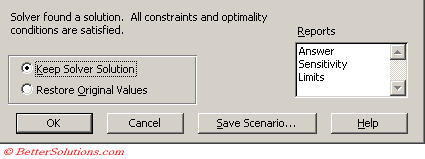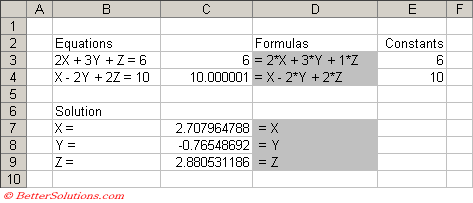### Important

After you apply the "Keep Solver Solution" option, you cannot undo the changes to the cells.
This can be very useful when used in combination with the financial worksheet functions.